# Linearity, Causality and Time-Invariance of a System¶

The notion of a system is central in digital communications and particularly system's theory. Abstractly, a system is defined as something that takes an input signal and produces an output signal by some transformation rule $Tr$. $$y(t)=Tr\{x(t)\}$$

Many relations in the real world can actually be understood as a system. Some examples include:

• You press a key on your keyboard, and the corresponding letter appears on your screen. What happens if you press two letters at the same time? Is this system "linear"?
• You speak into your microphone, and it converts your voice into electrical current. Hopefully this system does not introduce a lot of distortion.
• You inflate the tire of your bike. It responds with the pressure in the tire. The pressure can be seen as the summation of all the air that has flown into and out of the tire.

Let's take a more abstract example: A system can amplify the input signal, by doubling its amplitude: $$y(t)=2x(t)$$ Let us illustrate this very simple system with some code. As an example, we take the input signal $x(t)=\sin(t)$. Then, we can straight-forwardly implement the system as taking a function (a signal) and returning another time function (another signal):Run code interactively  Hide
x = lambda t: np.sin(t)  # the signal x(t)

def Tr(x):
return lambda t: 2*x(t)   # the IO relation y(t)=2*x(t)


Now, let's plot the input signal and the output signal over some time:

t = np.linspace(-5,5,1000)
plt.plot(t, x(t), label='Input $x(t)$')
y = Tr(x)  # send x through the system
plt.plot(t, y(t), label='Output $y(t)=2x(t)$')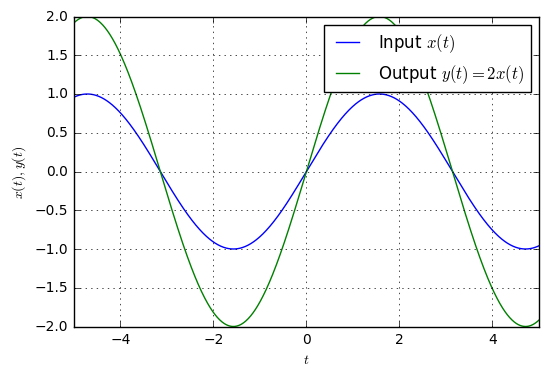Especially, three properties are critical characteristics of any system. Linear systems are most easy to analyze analytically, time-invariant systems allow to treat the systems input-output-relation independent of the absolute time and causal systems ensure that the system can be realized in real-time, since the system does not use information from the future.

Let us now go through each of these characteristics in more detail.

## Linear Systems¶

The rule of linearity is common among many mathematical and engineering aspects. Plainly, linearity describes that you can describe the effects of a system by separating the input signal into simple parts and using superposition at the output to restore the overall system output. Mathematically, we say that a system with transformation $Tr$ is linear if the following holds: $$Tr\{a\cdot x_1(t)+b\cdot x_2(t)\}=a\cdot Tr\{x_1(t)\} + b\cdot Tr\{x_2(t)\}.$$

Let us look at some systems to see if they are linear systems. First, let's define two input signals \begin{align}x_1(t)&=\sin(t)\\x_2(t)&=t, \text{periodic with period }0\leq t \leq 2\end{align} and write a function to check the linearity:

t = np.linspace(-10, 10, 1000)
x1 = lambda t: np.sin(t)
x2 = lambda t: (t % (2*np.pi))/np.pi

def checkLinearity(Tr, titleStr):
x1plusx2 = lambda t: x1(t)+x2(t)
plt.subplot(121)
plt.plot(t, x1(t), label=r'$x_1(t)$')
plt.plot(t, x2(t), label=r'$x_2(t)$')
plt.plot(t, x1plusx2(t), label=r'$x_1(t)+x_2(t)$')

plt.subplot(122)
plt.plot(t, Tr(x1)(t), label=r'$Tr\{x_1(t)\}$')
plt.plot(t, Tr(x2)(t), label=r'$Tr\{y_2(t)\}$')
plt.plot(t, Tr(x1)(t)+Tr(x2)(t), 'k--', lw=3, label=r'$Tr\{x_1(t)\}+Tr\{x_2(t)\}$')
plt.plot(t, Tr(x1plusx2)(t), label=r'$Tr\{x_1(t)+x_2(t)\}$')



Let's check the first system: $$y(t)=2x(t).$$

def Tr(x):
return lambda t: 2*x(t)
checkLinearity(Tr, '$y(t)=2x(t)$')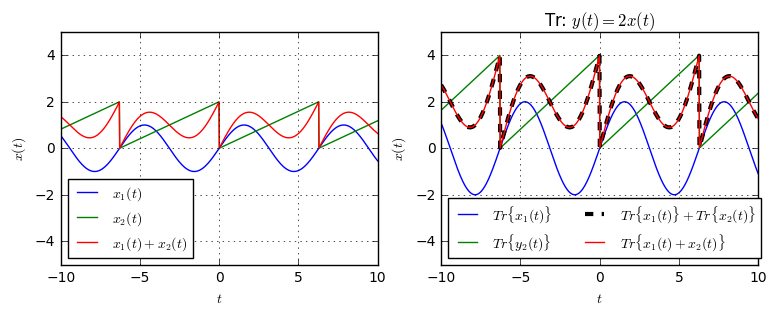Clearly, this system is linear, since the red and black curve overlap. (Actually, we cannot say yet that it's linear, because we have just found one example where it is linear. To really prove linearity, one would need to do this mathematically based on the input-output-relation. Despite not being too complicated, it is out of scope here).

Now, let's check another system, $$y(t)=x(t)^2.$$

def Tr(x):
return lambda t: x(t)**2
checkLinearity(Tr, '$y(t)=x(t)^2$')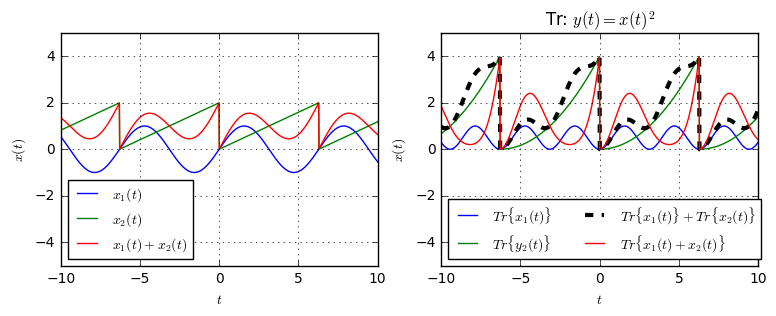Obviously, this system is not linear, since red and black do not overlap. (Here we can really say it is not linear, because we have found one example where the linearity condition does not hold).

Let's try a third system, $$y(t)=x(t-2).$$

def Tr(x):
return lambda t: x(t-2)
checkLinearity(Tr, '$y(t)=x(t-2)$')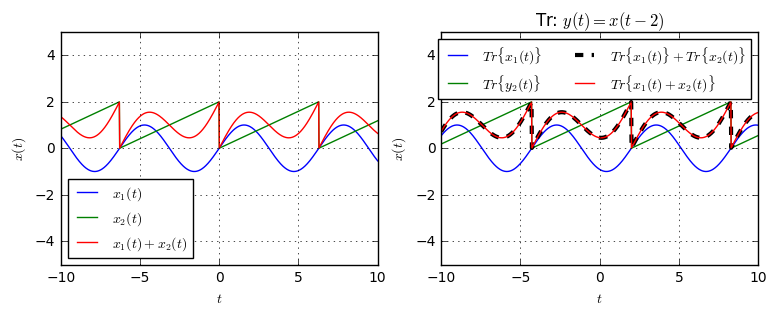Again, this system appears linear.

As the final system, what about this system? $$y(t)=x(t)-1$$

def Tr(x):
return lambda t: x(t)-1
checkLinearity(Tr, '$y(t)=x(t)-1$')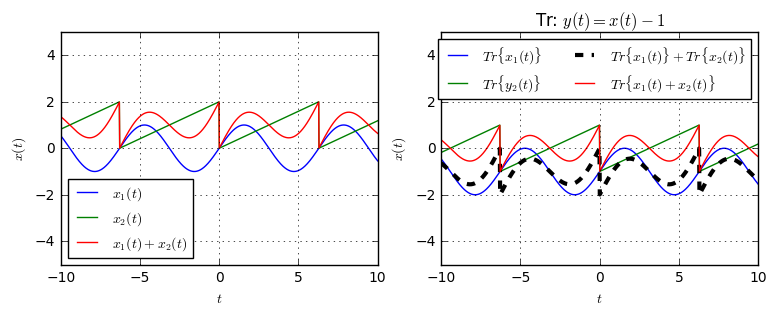Obviously, this system is non-linear.

For linear systems powerful mathematical tools have been developed. In particular, the superposition technique in conjunction with signal decompositions such as Fourier Series or Fourier Transform are valuable methods to analyze the input-output relation of a system.

How can we see, if a system is linear, just from looking at its transformation expression? As a rule of thumb, a system is linear, if the operations on the input signal are all linear and no signal-independent terms are contained. What are linear operations?

• Scaling of the input signal: $y(t)=ax(t)$
• Time-shifting the input signal $y(t)=x(t-a)$
• Scaling the argument of the signal $y(t)=x(at)$
• Combinations of the three above, e.g. $y(t)=2x(3(t-4))$
• Summations of terms that are linear, e.g. $y(t)=2x(t)+x(t-1)$

What are particularly non-linear operations?

• Multiplication of the signal with itself, i.e. $y(t)=x(t)\cdot x(t)$
• Applying any non-linear function to the signal, e.g. $y(t)=\sin(x(t))$
• Adding constant terms, which are independent of the signal, i.e. $y(t)=x(t)+a$

## Causal Systems¶

The property of causality is a requirement for a system to be realizable in reality. Causality means that the output of the system does not depend on future inputs, but only on past input. In particular, this means that if the input signal is zero for all $tor a predictable and input-independent signal for very fancy systems ) for all$t
t = np.linspace(-5, 5, 1000)
x = lambda t: (t>=0).astype(float)

def Tr1(x):
return lambda t: x(t-1)

def Tr2(x):
return lambda t: x(t+1)

def checkCausality(Tr):
plt.plot(t, x(t), label='Input $x(t)$')
plt.plot(t, Tr(x)(t), label=r'Output $y(t)=Tr\{x(t)\}$')

plt.subplot(121)
checkCausality(Tr1)

plt.subplot(122)
checkCausality(Tr2)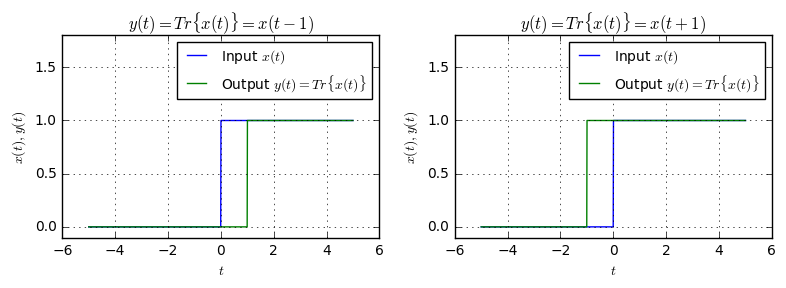Clearly, the first system, $y(t)=x(t-1)$ is a causal system, because its output is a time-delayed version of the original signal.

On the other hand, the second system $y(t)=x(t+1)$, is non-causal. This system returns a time-advanced version of the input signal. This means, that for example at the output time $t=0$, the system requires access to the value of the input signal at time $t=1$. Clearly, this is impossible in a realizable system, as nobody can look into the future.

## Time-Invariant Systems¶

A system is time-invariant if its output signal does not depend on the absolute time. In other words, if for some input signal $x(t)$ the output signal is $y_1(t)=Tr\{x(t)\}$, then a time-shift of the input signal creates a time-shift on the output signal, i.e. $y_2(t)=Tr\{x(t-t_0)\}=y_1(t-t_0)$.

A time-invariant system can be recognized from the fact that the transformation expression does not depend on the absolute time $t$, but $t$ is only used as an argument to the input functions. Let us look at some examples. First, let's define an exponential impulse as the input signal.

t = np.linspace(-2,4, 1000)
x = lambda t: np.exp(-t)*(t>=0).astype(float)

def showTimeInvariance(Tr):
plt.subplot(121)
plt.plot(t, x(t), label='$x(t)$')
plt.plot(t, x(t-0.5), label=r'$x(t-\frac{1}{2})$')
plt.plot(t, x(t-1), label=r'$x(t-1)$')

plt.subplot(122)
y1 = Tr(x)
y2 = Tr(lambda t: x(t-0.5))
y3 = Tr(lambda t: x(t-1))
plt.plot(t, y1(t), label=r'$Tr\{x(t)\}$')
plt.plot(t, y2(t), label=r'$Tr\{x(t-\frac{1}{2})\}$')
plt.plot(t, y3(t), label=r'$Tr\{x(t-1)\}$')


Now, let's see the output of the system $$y(t)=Tr\{x(t)\}=t\cdot x(t)$$ for the input of a time-shifted exponential impulse:

def Tr(x):
return lambda t: t*x(t)
showTimeInvariance(Tr)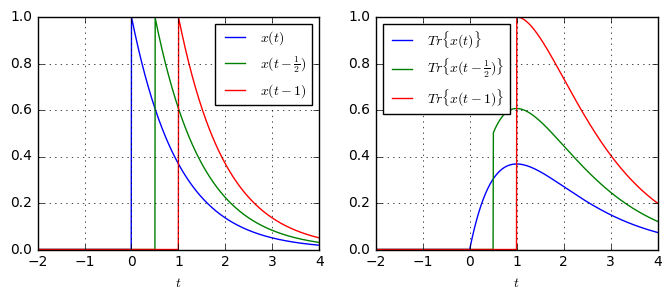Clearly, the system is not time-invariant: When the inputs of the system are time-shifted exponential impulses, the outputs of the system are not just time-shifted versions of each other. Hence, the system is not time-invariant, but it is time-variant.

As a second example, let's have a look at the system $$y(t)=Tr\{x(t)\}=x(t)^2.$$

def Tr(x):
return lambda t: x(t)**2
showTimeInvariance(Tr)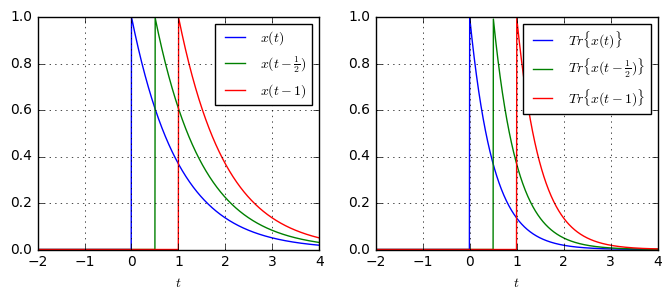This system is time-invariant, since the output signals of the system are just time-shifted versions of each others, when the input are time-shifted versions of each other.

Finally, let's look at a third example of a system, which is given by $$y(t)=Tr\{x(t)\}=x(2t).$$

def Tr(x):
return lambda t: x(2*t)
showTimeInvariance(Tr)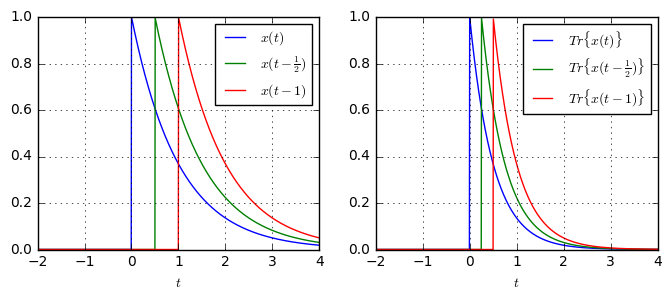Is this system time-invariant? At first glance, it appears to be: The inputs are time-shifted to each other, the outputs are time-shifted to each other. But: The definition of time-invariance states: $$Tr\{x(t)\}=y(t) \Rightarrow Tr\{x(t-t_0)\}=y(t-t_0)$$ I.e. a time-shift by $t_0$ of the input signal creates the same time-shift by $t_0$ at the output.

Let's look once again at the system above: The time-shift between the red and blue input is equals $t_0=1$. However, the time-shift between the blue and red output signals is just $0.5$. Hence, even though the outputs are time-shifted versions of each other, their amount of time-shift is not equal to the input shift. Therefore, this system is not time-invariant.

## Relation between the properties¶

We have analyzed three properties of a system:

• Linearity
• Causality
• Time-invariance

How do these properties relate to each other? Essentially, these properties are independent of each other and they can appear in any combination. Some examples:

\begin{align} y(t)&=Tr\{x(t)\}=x(t)^2 && \text{non-linear, causal, time-invariant}\\ y(t)&=Tr\{x(t)\}=t\cdot x(t) && \text{linear, causal, time-variant}\\ y(t)&=Tr\{x(t)\}=x(t+1) && \text{linear, non-causal, time-invariant}\\ y(t)&=Tr\{x(t)\}=\sin(x(t))\cdot sin(t) && \text{non-linear, causal, time-variant} \end{align}

We could go on for ever and find examples for each combination of properties. However, one particular combination is especially important in signal processing: The class of Linear Time-Invariant (LTI) systems. All these systems can be described by their response to a Dirac input, which is called the impulse response. The class of LTI systems is so important that it deserves a dedicated article, which I'll write soon. Subscribe to the newsletter to be first to know about new content!

## Summary¶

We have described three properties of a system, which can be recognized by the following rules of thumb:

• Linearity: A system is linear, if it only consists of linear operations, such as: scaling, time-shift, summations of scaled and time-shifted input signals. Any other operation is likely non-linear.
• Causality: A system is causal, if for any time $t_0$, the output of the system is completely defined by the values of the input signal for times \$t
• Time-Invariance: If the input to a time-invariant system is shifted in time, its output remains the same signal, but is shifted equally in time.Do you have questions or comments? Let's dicuss below!

### Related posts

DSPIllustrations.com is a participant in the Amazon Services LLC Associates Program, an affiliate advertising program designed to provide a means for sites to earn advertising fees by advertising and linking to amazon.com, amazon.de, amazon.co.uk, amazon.it.